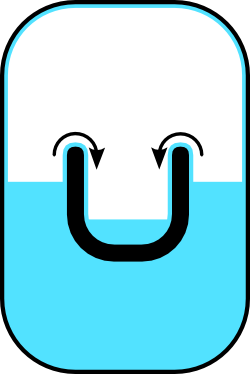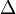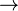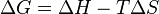# Absolute zeroNear absolute zero (0 K), matter exhibits strange properties. For example, below a temperature of 2.1768 K (the "lambda point"), helium is a superfluid that "creeps" along surfaces against the force of gravity and forms a film ("Rollin film"). Here, the Rollin film covers the interior of the larger, sealed container. After a short while, the levels of helium in the outer and inner containers will equalize.

Absolute zero is the lowest possible temperature, such that nothing could be colder and no heat energy remains in the material being examined. At this temperature, the molecules stop moving, with minimal or no vibrational motion, retaining only quantum mechanical, zero-point energy-induced particle motion.

By international agreement, absolute zero is defined as precisely:

• 0 K on the Kelvin scale, which is a thermodynamic temperature (or absolute temperature) scale, and
• –273.15 °C on the Celsius scale.

In addition, absolute zero is precisely equivalent to:

• 0 °R on the Rankine scale, a lesser-used thermodynamic temperature scale, and
• –459.67 °F on the Fahrenheit scale.

The ratios of two absolute temperatures, T2/T1, are the same in all scales.

Although scientists working in the field of cryogenics cannot fully achieve a state of “zero” heat energy in a substance, they have made great advances in achieving temperatures that draw ever closer to absolute zero, where matter exhibits odd quantum effects. The emergence of dramatically new properties of substances near absolute zero illustrates the fact that under extreme conditions, simple extrapolations of the known laws of physics and chemistry become invalid. These experiments have time and again shown up the limitations of existing theory and pointed the way to a more profound understanding of matter.

## Record cold temperatures approaching absolute zero

It can be shown from the laws of thermodynamics that absolute zero can never be achieved artificially, though it is possible to reach temperatures arbitrarily close to it through the use of cryocoolers. This is the same principle that ensures no machine can be 100 percent efficient.

At very low temperatures in the vicinity of absolute zero, matter exhibits many unusual properties including superconductivity, superfluidity, and Bose-Einstein condensation. To study such phenomena, scientists have worked to obtain ever lower temperatures.

• In 1994, the National Institute of Standards and Technology (NIST) achieved a record cold temperature of 700 nK (nanokelvin, or 10-9  K).
• In September 2003, researchers at the Massachusetts Institute of Technology announced a record cold temperature of 450 picokelvin (pK), or 4.5 × 10-10  K, in a Bose-Einstein condensate of sodium atoms. This was performed by Wolfgang Ketterle and colleagues at MIT.
• As of February 2003, the Boomerang Nebula, with a temperature of 1.15  K, is the coldest place known outside a laboratory. The nebula is five thousand light-years from Earth and is in the constellation Centaurus.
• As of November 2000, nuclear spin temperatures below 100 pK were reported for an experiment at the Low Temperature Lab of the Helsinki University of Technology. This, however, was the temperature of one particular type of motion—a quantum property called nuclear spin—not the overall average thermodynamic temperature for all possible degrees of freedom.

## Thermodynamics near absolute zero

### Molecular motion in crystals

At 0 K, (nearly) all molecular motion ceases and the change in entropy is zero ($\Delta$S = 0) for any adiabatic process. Pure substances can (ideally) form perfect crystals as the temperature approaches absolute zero (T$\rightarrow$0). According to Planck's strong form of the third law of thermodynamics, the entropy (S) of a perfect crystal vanishes at absolute zero. This prediction, however, cannot be valid if the lowest energy state for the substance is degenerate—that is, if the substance can exist in more than one microstates of energy.

The original Nernst heat theorem makes the weaker and less controversial claim that the change of entropy (ΔS) for any isothermal process approaches zero as the temperature approaches absolute zero (T → 0):$\lim_{T \to 0} \Delta S = 0$

This equation implies that the entropy of a perfect crystal simply approaches a constant value. "The Nernst postulate identifies the isotherm T = 0 as coincident with the adiabat S = 0, although other isotherms and adiabats are distinct. As no two adiabats intersect, no other adiabat can intersect the T = 0 isotherm. Consequently no adiabatic process initiated at nonzero temperature can lead to zero temperature” (Callen, 189-190). An even stronger assertion is that "it is impossible by any procedure to reduce the temperature of a system to zero in a finite number of operations" (Guggenheim, 157).

A perfect crystal is one in which the internal lattice structure extends uninterrupted in all directions. The perfect order can be represented by translational symmetry along three (not usually orthogonal) axes. Every lattice element of the structure is in its proper place, whether it is a single atom or molecular grouping. For substances that have two (or more) stable crystalline forms, such as diamond and graphite for carbon, there is a kind of "chemical degeneracy." The question remains whether both can have zero entropy at T = 0 even though each is perfectly ordered.

Perfect crystals never occur in practice. Imperfections, and even entire amorphous materials, simply get "frozen in" at low temperatures, so that transitions to more stable states do not occur.

### Drop in specific heat and various thermodynamic quantities

Using the Debye model, the specific heat and entropy of a pure crystal are proportional to T 3, while the enthalpy (H) and chemical potential are proportional to T 4 (Guggenheim, 111). Based on this model, one may predict that as the temperature approaches absolute zero, these quantities drop toward their limiting values and approach with zero slopes.

In the case of specific heats at least, the limiting value itself is zero, as borne out by experiments conducted below 10 K. Even the less detailed Einstein model shows this curious drop in specific heats. These models indicate that all specific heats vanish at absolute zero, not just those of crystals. Likewise for the coefficient of thermal expansion. Maxwell's relations show that various other quantities also vanish. These phenomena were unanticipated.

### Changes during chemical reactions

The relationship between changes in Gibbs free energy (ΔG), enthalpy (ΔH), and entropy (ΔS) is given by the following equation:$\Delta G = \Delta H - T \Delta S \,$

From this equation, it follows that as T decreases, ΔG and ΔH approach each other (so long as ΔS remains unchanged). Experimentally, it is found that most chemical reactions are exothermic in the direction they are going—that is, they release heat as they move toward thermodynamic equilibrium. For an exothermic reaction, ΔH has a negative value (ΔH < 0). Even at room temperature, T is low enough so that the fact that (ΔG)T,P < 0 (usually) implies that ΔH < 0. On the other hand, if the reaction were moving in the opposite direction, it would absorb heat, and ΔH would have a positive value.

Moreover, the slopes of the temperature derivatives of ΔG and ΔH converge and are equal to zero at T = 0. Consequently, ΔG and ΔH are nearly the same over a considerable range of temperatures. This relationship between ΔG and ΔH justifies the approximate empirical principle of Thomsen and Berthelot, which says that the equilibrium state to which a system proceeds is the one that evolves the greatest amount of heat. In other words, an actual process is (usually) the most exothermic one (Callen, 186-187).

## Negative temperatures

Certain semi-isolated systems (for example a system of non-interacting spins in a magnetic field) can achieve "negative" temperatures. They, however, are not actually colder than absolute zero. They can be thought of as "hotter than T=∞", as energy will flow from a negative temperature system to any other system with positive temperature upon contact.